# 基于列车制动综合试验台的黏着系数测试方法研究Research on Adhesive Coefficient Test Method Based on Train Brake Integrated Test-Bed

• 全文下载: PDF(1038KB)    PP.16-22   DOI: 10.12677/OJTT.2020.91003
• 下载量: 58  浏览量: 89

In this paper, the 1:1 train brake integrated test-bed is taken as the research object, and the test methods of the characteristic parameters related to the adhesive characteristics under the brak-ing condition are analyzed in detail, including the slip ratio, adhesive force and positive pressure. Finally, the test-bed was used to carry out the repeated test under the same working condition, and the relationship between the adhesion coefficient and slip ratio was obtained through data sorting and analysis. It was confirmed that the 1:1 train brake integrated test-bed can meet the test of the adhesion coefficient between the wheel and rail during the braking process.

1. 引言

2. 制动系统综合试验台简介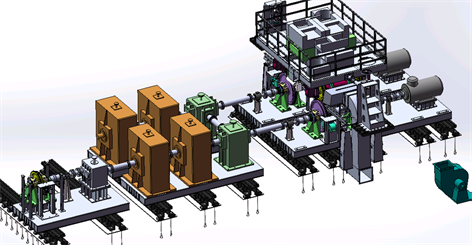Figure 1. 3D map of the train brake integrated test-bedFigure 2. Test-bed layout

3. 试验原理

3.1. 参数测量原理

$\mu =\frac{F}{N}$ (1)

$\varsigma =\frac{v-w\cdot r}{v}$ (2)

3.2. 特征参数测算方案

3.2.1. 滑移率测算方案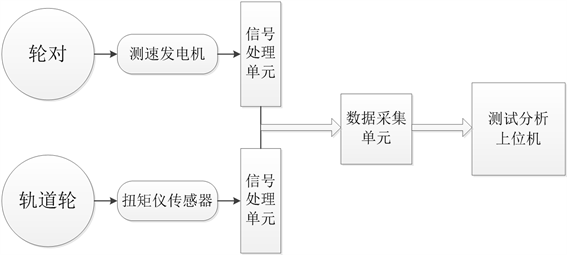Figure 3. Slip rate measurement graph

3.2.2. 黏着力测算方案

${T}_{1}+{T}_{2}-T=2\cdot {I}_{R}\cdot \frac{\stackrel{˙}{v}}{R}$ (3)

$F=\frac{T}{R}=\frac{{T}_{1}+{T}_{2}-2\cdot {I}_{R}\cdot \frac{\stackrel{˙}{v}}{R}}{R}$ (4)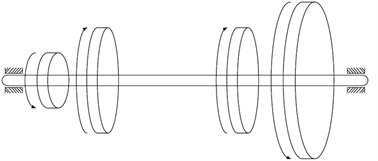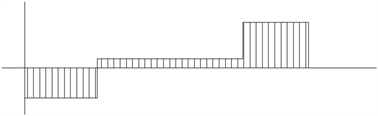Figure 4. Brake shaft cross-sectional torque graph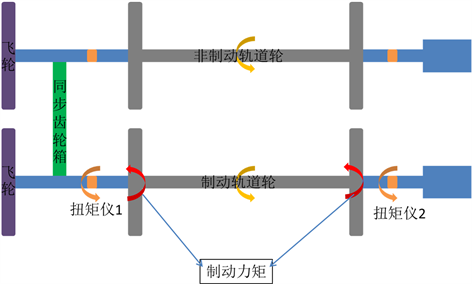Figure 5. Power force analysis

3.2.3. 正压力测算方案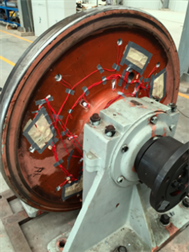Figure 6. Installation diagram of positive pressure test instruments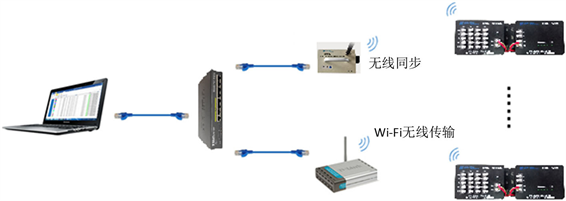Figure 7. Connection-mode of the computer and the instrument

4. 验证性试验及其结果

a) 用两台牵引电机将轨道轮和车轮牵引至速度100 km/h，保持洒水500 ml/min；

b) 开始对一根轴施加制动，设定制动缸压力为最大600 KPa，实时测算黏着系数和滑移率；

c) 当滑移率达到临界值(设定的最大值)时防滑阀自动排气停止制动；

d) 重复1~3试验步骤多次，完成同一工况下的多次重复试验。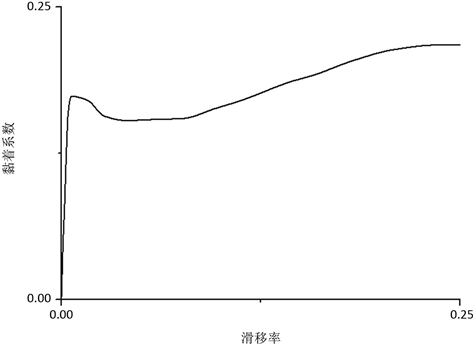Figure 8. Relationship between adhesion coefficient and slip rate

5. 结论

  金学松, 郭俊, 肖新标, 等. 高速列车安全运行研究的关键科学问题[J]. 工程力学, 2009(S2): 8-22.  李宁洲. 机车黏着智能优化控制研究[D]: [博士学位论文]. 成都: 西南交通大学, 2015.  史来诚. 基于黏着与滑模控制的高速列车制动方法研究[D]: [硕士学位论文]. 株洲: 湖南工业大学, 2019.  王文健, 张鸿斐, 刘启跃. 高速轮轨黏着机制进展及模拟试验研究[J]. 润滑与密封, 2011, 36(11): 105-108.  Zhang, W., Chen, J., Wu, X., et al. (2002) Wheel/Rail Adhesion and Analysis by Using Full Scale Roller Rig. Wear, 253, 82-88. https://doi.org/10.1016/S0043-1648(02)00086-8  Chen, H., Ban, T., Ishida, M., et al. (2008) Experimental Investigation of Influential Factors on Adhesion between Wheel and Rail Under Wet Conditions. Wear, 265, 1504-1511. https://doi.org/10.1016/j.wear.2008.02.034  黄辉. 轮轨垂向力连续测量方法研究[D]: [硕士学位论文]. 南昌: 华东交通大学, 2016.  乔立山, 王玉兰, 曾锦光. 实验数据处理中曲线拟合方法探讨[J]. 成都理工大学学报(自然科学版), 2004, 31(1): 91-95.